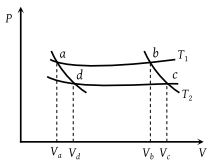# NEET Physics Thermodynamics Questions Solved

In the following P-V diagram two adiabatics cut two isothermals at temperatures T1 and T2 (fig.). The value of $\frac{{V}_{a}}{{V}_{d}}$ will be(1) $\frac{{V}_{b}}{{V}_{c}}$

(2) $\frac{{V}_{c}}{{V}_{b}}$

(3) $\frac{{V}_{d}}{{V}_{a}}$

(4) VbVc

Concept Videos :-

#3 | Thermodynamic Processes
#4 | Isobaric Process
#5 | Isochoric & Isothermal Process
#6 | Interconversion of Graphs
#7 | Solved Example: 1
#8 | Solved Example: 2